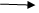# LAMBDA EXPRESION IN LINQ

21 September, 2018

Ratings

## LAMBDA EXPRESSION IN LINQLambda expression are used to work on same group of data in general used with agitate function. Def :- A lambda expression is a function with out a name calculates and returns a single value.

• Lambda Expression is represented by lambda  operator  (=>) to be read as “Goes to”
• Lambda Expression can be device as 2 categories
1. Expression lambda
2. Statement lambda

### Expression lambda :-

Input parametersExpression Statement lambda :- Input parameters{ Expression; }

### Example with Expression Lambdas :-

```Namespace CA Sample 2
{
Class Example2
{
Static void Main ()
{
List <int> L1 = new Lint  <int> {1,2,3,4,5,6,7,8,9,10};
Int c = L1.count  (x =>x >4);
Int E count  = L1.count  (x => xy = z ==0);
C.W.L (“Count of values grater than 4 is :- ” + c);
C.W.L (“Count of values Even numbers  is :- ” + E count);
}
}
}```

### Output :-

Count of values grater than 4 is :-    6 Count of values Even numbers are  :-  5

```At TekSlate, we offer resources that help you in learning various IT courses.
We avail both written material and demo video tutorials.
To gain in-depth knowledge and be on par with practical experience, then explore ASP.net Training Videos.```

Example  to implement  lambda expression on employee class :- Example to count particular dept no and print on the column :-

```Namespace CA Sample 2
{
Class Example3
{
Static void Main ()
{
List <Employee> emp list = new list <Employee> ()
{
New  employee  (P emp id = 101, P Ename = “N”, Pdesigen = “pl”, p Doj = new date (2012,03,02),p salary = 1200, pdeptno =10);
New  employee  (P emp id = 102, P Ename = “R”, Pdesigen = “tl”, p Doj = new date (2012,04,01),p salary = 1300, pdeptno =20);
New  employee  (P emp id = 103, P Ename = “P”, Pdesigen = “ds”, p Doj = new date (2012,05,03),p salary = 1400, pdeptno =30);
};
Var  R = from  x in Emplist. Select x. p dept no;
Int c1 = R.count  (I => i. 10);
Int c2 = R.count  (I => i. 20);
Int c3 = R.count  (I => i. 30);
C.W.L   (“Deptno /t : count /n………………”);
C.W.L (“10/t”  + C1);
C.W.L (“20/t”  + C2);
C.W.L (“30/t”  + C3);
C.W.L (“total/t”  + R.count ());
}
}
}```
For indepth understanding click on